Next:9.10 Merge SortUp:9. Sorting MethodsPrevious:9.8.2 Result 2: Lower Bound on Average Case Complexity

• Here we use some special information about the keys and design sorting algorithms which beat the O(nlog n) lower bound for comparison-based sorting methods.
• Consider sorting n integers in the range 0 to n2 - 1. We do it in two phases.
• Phase 1:
We use n bins, one for each of the integers 0, 1, ... , n - 1. We place each integer i on the list to be sorted into the bin numbered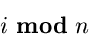Each bin will then contain a list of integers leaving the same remainder when divided by n.

At the end, we concatenate the bins in order to obtain a list L.

Phase 2:
The integers on the list L are redistributed into bins, but using the bin selection function: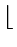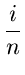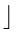Now append integers to the ends of lists. Finally, concatenate the lists to get the final sorted sequence.
Example
n = 10
Initial list : 36, 9, 0, 25, 1, 49, 64, 16, 81, 4
Phase 1 : bin = i mod 10

= right most digit of i
Bin

0
 0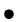1
 1
 812
3
4
 64
 45
 25

6
 36
 167
8
9
 9
 49Concatenation would now yield the list:

L: 0, 1, 81, 64, 4, 25, 36, 16, 9, 49

Phase 2 : bin =i/10= right most digit of i
Bin

0
 0
 1
 4
 9

1
 16

2
 25

3
 36

4
 49

5

6
 64

7

8
 81

9

The concatenation now yields:

0, 1, 4, 9, 25, 36, 49, 64, 81

In general, assume that the key-type consists of k components f1, f2, ... , fk, of type t1, t2, ... , tk.

Suppose it is required to sort the records in lexicographic order of their keys. That is

(a1, a2,..., ak) < (b1, b2,..., bk)
if one of the following holds:
 1. a1 < b1 2. a1 = b1;a2 < b2 3. a1 = b1;a2 = b2;a3 < b3 . . . k. a1 = b1;...;ak - 1 = bk - 1;ak < bk

Radix sort proceeds in the following way.

First binsort all records first on fk, the least significant digit, then concatenate the bins lowest value first.

Then binsort the above concatenated list on fk - 1 and then concatenate the bins lowest value first.

In general, after binsorting on fk, fk - 1,..., fi, the records will appear in lexicographic order if the key consisted of only the fieldsfi,..., fk.

/ * Sorts a list A of n records with keys consisting of fields

f1,..., fk of types t1,..., tk. The function uses k

arrays B1,..., Bk of type array [ti] of list-type, i = 1,..., k,

where list-type is a linked list of records */

{

for(i = k;i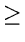1;i - -)

{

for (each value v of type ti)

make Bi[v] empty; /* clear bins */

for (each value r on list A)

move r from A onto the end of bin Bi[v],

where v is the value of the field fi of the key of r;

for (each value v of type ti from lowest to highest)

concatenate Bi[v] onto the end of A

}

}

• Elements to be sorted are presented in the form of a linked list obviating the need for copying a record. We just move records from one list to another.
• For concatenation to be done quickly, we need pointers to the end of lists.
• Inner loop 1 takes O(si) time where si = number of different values of type ti
• Inner loop 2 takes O(n) time
• Inner loop 3 times O(si) time

• Thus the total time

 =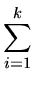O(si + n) = O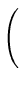kn +si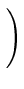= On +si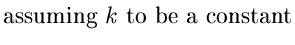• For example, if keys are integers in the range 0 to nk - 1, then we can view the integers as radix - n integers each k digits long. Then tihas range  ...  n - 1 for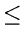ik.

• Thus si = n and total running time is O(n)

• similarly, if keys are character strings of length k, for constant k, then si = 128 (say) for i = 1,..., k and again we get the total running time as O(n).Next:9.10 Merge SortUp:9. Sorting MethodsPrevious:9.8.2 Result 2: Lower Bound on Average Case Complexity
eEL,CSA_Dept,IISc,Bangalore